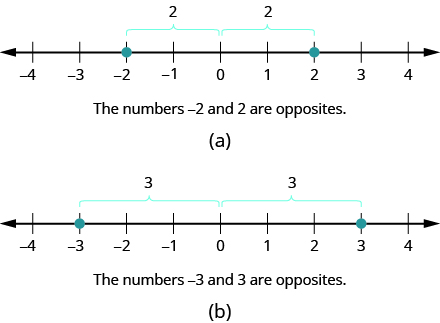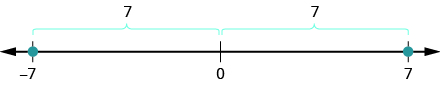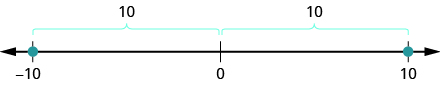## Notation and Definition of the Set of Integers

### Learning Outcomes

• Write the opposite of a given number
• Use correct notation to indicate the opposite of a number
• Identify the elements of the set of integers as the counting numbers, their opposites, and zero

On the number line, the negative numbers are a mirror image of the positive numbers with zero in the middle. Because the numbers $2$ and $-2$ are the same distance from zero, they are called opposites. The opposite of $2$ is $-2$, and the opposite of $-2$ is $2$ as shown in figure(a). Similarly, $3$ and $-3$ are opposites as shown in figure(b).### Opposite

The opposite of a number is the number that is the same distance from zero on the number line, but on the opposite side of zero.

### example

Find the opposite of each number:

1. $7$

2. $-10$

Solution:

1. The number $-7$ is the same distance from $0$ as $7$, but on the opposite side of $0$. So $-7$ is the opposite of $7$ as shown below.2. The number $10$ is the same distance from $0$ as $-10$ , but on the opposite side of $0$. So $10$ is the opposite of $-10$ as shown below.### try it

The video below shows more examples of how to find the opposite of an integer.

### Opposite Notation

Just as the same word in English can have different meanings, the same symbol in algebra can have different meanings. The specific meaning becomes clear by looking at how it is used. You have seen the symbol “$-$” in three different ways.

 $10 - 4$ Between two numbers, the symbol indicates the operation of subtraction. We read $10 - 4$ as 10 minus $4$ . $-8$ In front of a number, the symbol indicates a negative number. We read $-8$ as negative eight. $-x$ In front of a variable or a number, it indicates the opposite. We read $-x$ as the opposite of $x$ . $-\left(-2\right)$ Here we have two signs. The sign in the parentheses indicates that the number is negative 2.The sign outside the parentheses indicates the opposite. We read $-\left(-2\right)$ as the opposite of $-2$, which is $2$.

### Opposite Notation

$-a$ means the opposite of the number $a$
The notation $-a$ is read the opposite of $a$.

### example

Simplify: $-\left(-6\right)$.

### Integers

The set of counting numbers, their opposites, and $0$ is the set of integers.
Integers are counting numbers, their opposites, and zero.

$\dots{-3,-2,-1,0,1,2,3}\dots$

We must be very careful with the signs when evaluating the opposite of a variable.

### example

Evaluate $-x:$

1. When $x=8$
2. When $x=-8$.